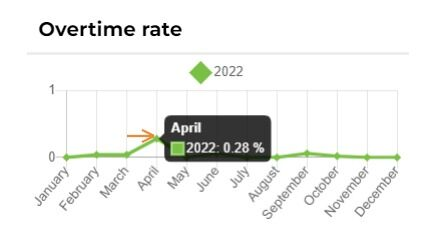# Rates and trends - Overtime rateJean-Simon Bolduc
• Updated

1. The overtime rate results provide analysis of the overtime ratio versus the regular hours ratio.

2. The overtime rate is calculated as follows: The number of overtime hours divided by the number of regular hours to be worked multiplied by 1000.

3. You can view the total for each month by placing your cursor over the desired month.Related to